2019-11-15

## 什么是表达式

``SELECT (count * price) AS sum FROM orders WHERE order_id < 100 ``

## RPN 表达式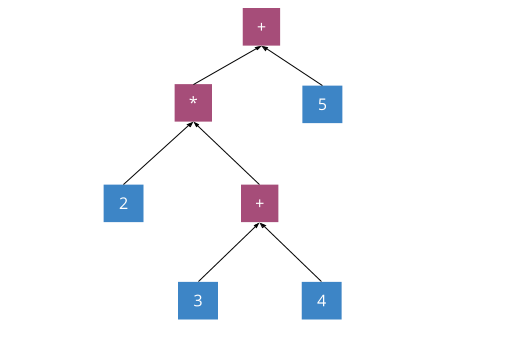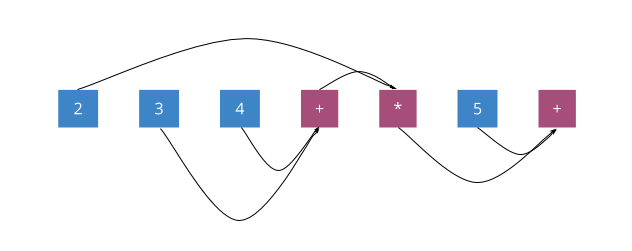## 构建 RPN 表达式Coprocessor 中表达式的定义：

``````/// An expression in Reverse Polish notation, which is simply a list of RPN expression nodes.
///
/// You may want to build it using RpnExpressionBuilder.
#[derive(Debug, Clone)]
pub struct RpnExpression(Vec<RpnExpressionNode>);
/// A type for each node in the RPN expression list.
#[derive(Debug, Clone)]
pub enum RpnExpressionNode {
/// Represents a function call.
FnCall {
func_meta: RpnFnMeta,
args_len: usize,
field_type: FieldType,
implicit_args: Vec<ScalarValue>,
},
/// Represents a scalar constant value.
Constant {
value: ScalarValue,
field_type: FieldType,
},
/// Represents a reference to a column in the columns specified in evaluation.
ColumnRef { offset: usize },
}``````

## 执行 RPN 表达式

``````// A type for each node in the RPN evaluation stack. It can be one of a scalar value node or a
/// vector value node. The vector value node can be either an owned vector value or a reference.
#[derive(Debug)]
pub enum RpnStackNode<'a> {
/// Represents a scalar value. Comes from a constant node in expression list.
Scalar {
value: &'a ScalarValue,
field_type: &'a FieldType,
},
/// Represents a vector value. Comes from a column reference or evaluated result.
Vector {
value: RpnStackNodeVectorValue<'a>,
field_type: &'a FieldType,
},
}``````

• 标量：由 `Constant` 生成。
• 向量：执行 `ColumnRe f` 生成，或是 `FnCall` 调用返回的结果。

``````/// Represents a vector value node in the RPN stack.
///
/// It can be either an owned node or a reference node.
///
/// When node comes from a column reference, it is a reference node (both value and field_type
/// are references).
///
/// When nodes comes from an evaluated result, it is an owned node.
#[derive(Debug)]
pub enum RpnStackNodeVectorValue<'a> {
Generated {
physical_value: VectorValue,
logical_rows: Arc<[usize]>,
},
Ref {
physical_value: &'a VectorValue,
logical_rows: &'a [usize],
},
}``````

1. 首先我们准备好一个栈结构：2. 接着逐一遍历表达式，第一个取出的是 `ColumnRef`，我们取出输入 Selection 算子的数据中对应 offset 的列的向量数据，并将向量压入栈：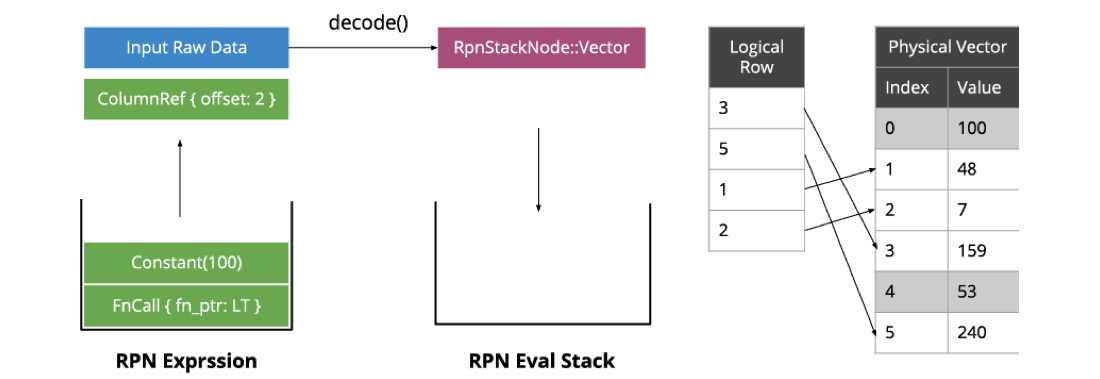3. 接着是 `Constant`，转化为标量然后压入栈：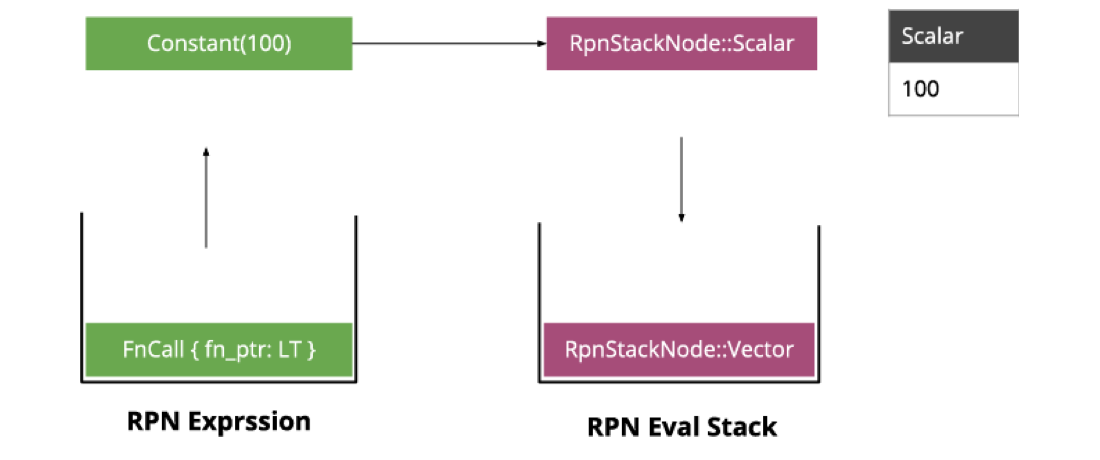4. 最后一个是 `LT` 运算符，它需要两个入参，因此我们从栈中弹出两个值作为参数调用 `LT``LT` 会生成一个新的向量，将结果压入栈：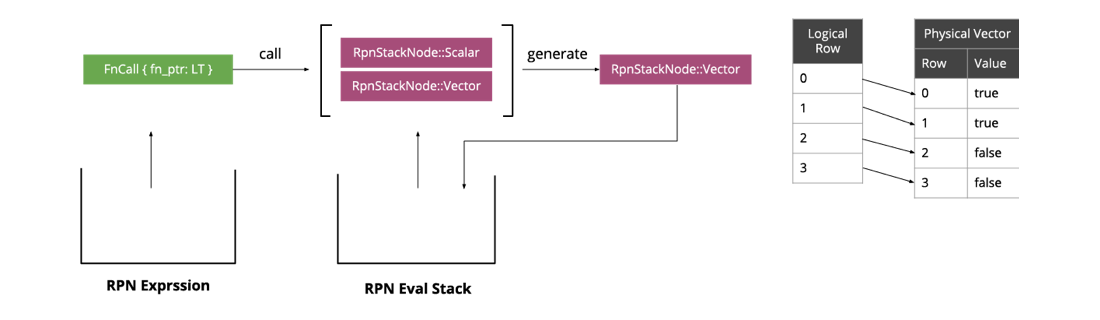5. 最后留在栈里的就是表达式的执行结果。6. Selection 算子根据结果的布尔值过滤原输入的逻辑索引：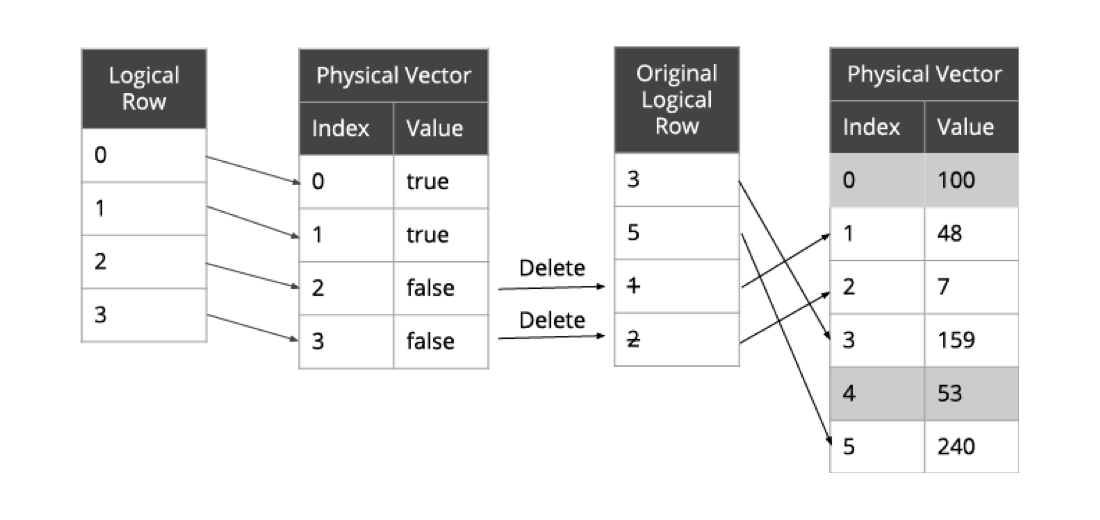7. 这样就间接的过滤出有效数据而不用改变 Physical Vector：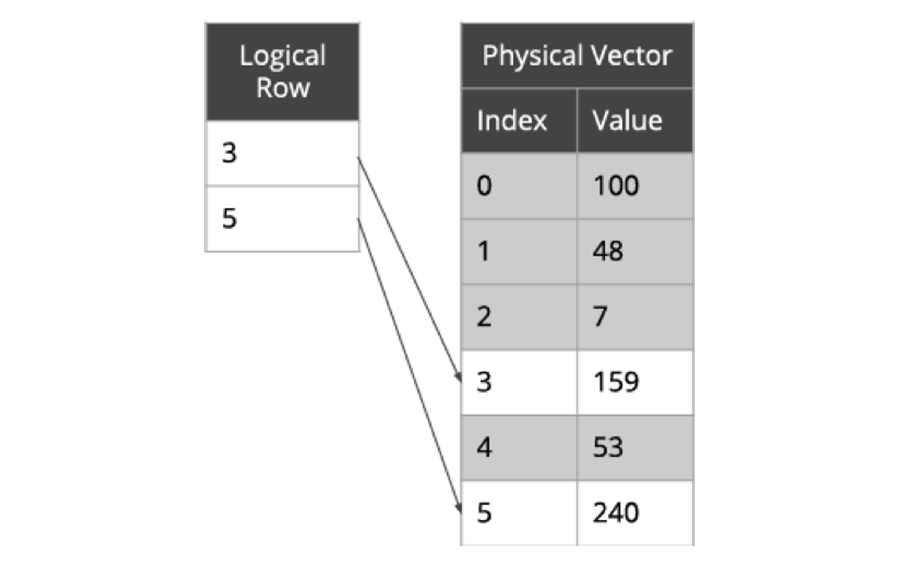## 实现 RPN 表达式函数

``````#[rpn_fn]
#[inline]
pub fn int_plus_int(
lhs: &Option<Int>,
rhs: &Option<Int>,
) -> Result<Option<Int>> {
if let (Some(lhs), Some(rhs)) = (arg0, arg1) {
.ok_or_else(|| Error::overflow("BIGINT", &format!("({} + {})", lhs, rhs)).into())
.map(Some)
} else {
Ok(None)
}
}``````

`#[rpn_fn]` 宏会分析这个操作符定义的参数数量和类型，自动生成既可以处理标量也可以处理向量的 `int_plus_int_fn_meta()`，这个函数将可以放进 `FnCall` 被用于表达式计算：

``````pub fn int_plus_int_fn_meta(
_ctx: &mut EvalContext,
output_rows: usize,
args: &[RpnStackNode<'_>],
_extra: &mut RpnFnCallExtra<'_>,
) -> Result<VectorValue>
{
assert!(args.len() >= 2);
let lhs = args;
let rhs = args;
let mut result: Vec<Int> = Vec::with_capacity(output_rows);
match lhs {
RpnStackNode::Scalar { value: ScalarValue::Int(lhs) , .. } => {
match rhs {
RpnStackNode::Scalar { value: ScalarValue::Int(rhs) , .. } => {
let value = int_plus_int(lhs, rhs);
result.push(result);
}
RpnStackNode::Vector { value: VectorValue::Int(rhs_vector) , .. } => {
for rhs_row in rhs_vector.logical_rows() {
let rhs = rhs_vector.physical_value[rhs_row];
let value = int_plus_int(lhs, rhs);
result.push(result);
}
}
_ => panic!("invalid expression")
}
}
RpnStackNode::Vector { value: VectorValue::Int(lhs_vector) , .. } => {
match rhs {
RpnStackNode::Scalar { value: ScalarValue::Int(rhs) , .. } => {
for lhs in lhs_vector {
let value = int_plus_int(lhs, rhs);
result.push(result);
}
}
RpnStackNode::Vector { value: VectorValue::Int(rhs_vector) , .. } => {
for (lhs, rhs) in lhs_vector.logical_rows().iter().zip(rhs_vector.logical_rows()) {
let lhs = lhs_vector.physical_value[lhs_row];
let rhs = rhs_vector.physical_value[rhs_row];
let value = int_plus_int(lhs, rhs);
result.push(result);
}
}
_ => panic!("invalid expression")
}
}
_ => panic!("invalid expression")
}
result
}``````